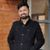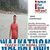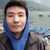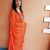# valuation for a perpetual bond, preferred stock, and common stock with constant dividend

Explain why the valuation for a perpetual bond, preferred stock, and common stock with constant dividend payments (zero growth) are virtually identical?

This post was part of TyroCity discussion forumAngel Paudel

Perpetual bonds are those type of bonds which don’t have any maturity date. Its price is the PV of its current interest payment with no finite end date. It thus pays the interest rate but with no finite end date, the only way to get back the face amount of the bond is by selling it (Hatchondo & Martinez, 2009). Preferred stocks on the other hand often always pays a fixed dividend. This fixed payout of dividends in a way disowns the holders from the benefit of the organizational growth. These types of stock go upto perpetuity as well. These stockholders are paid the dividends before the common stockholders however they don’t have the voting rights. Common stocks come with the voting rights meaning the one with majority shares can even control the company. Higher earning of the company can make the stock price of the company go up. Both stock price and interest rate can go up in the same direction in this form of investment/holdings. These type of stocks aren’t callable unlike the other two. They do continue upto perpetuity.

The dividend growth model is used as a way to get the valuation of the company’s bond or stock without any constraint to the outer industrial conditions (Barnes, 2015). Any investor expects to get the return out of their investment when they allow the business to use their money to meet the financial need of the company. The payment that the company makes to the investor at a fixed period of time is called as a dividend. The zero growth of dividend means that the stock will yield constant return year by year. This model is thus considered to be more of a theoretical approach rather than practically feasible. As all the three values we’ve continue upto perpetuity, the present value of stock with zero growth can be calculated using the formula dividends per period divided by the required rate of return of each period (Miller & Modigliani, 1961). They yield the same return which is always fixed and with the constant dividends, return is fixed for as long as the company is in existence. Also, from the above formula, both the parameters are same for all three - perpetual bond, preferred stock and common stock and thus the present value is obtained to be same (considering both the dividend and the required rate is same for the same level of investment).

References

Barnes, P. (2015). Dividend Growth Model. Wiley Encyclopedia Of Management , 1-4.

Hatchondo, J., & Martinez, L. (2009). Long-duration bonds and sovereign defaults. Journal Of International Economics , 79 (1), 117-125.

Miller, M., & Modigliani, F. (1961). Dividend Policy, Growth, and the Valuation of Shares. The Journal Of Business , 34 (4), 411-413.A bond which is issued without a finite maturity period is called perpetual bond. A perpetual bond or promise to pay interest identifitely and there is no contractual obligation to repay the principal. The bond is also called irredeemable which are rarely found in practice.

The value of a perpetual bond with simply equal to the present value of an infinite stream of interest payment (INT) which is discounted at investor’s required rate of return (Kd).

The value of perpetual bond is determined as follow:

Bo = INT/ Kd

For example, A bond of Rs. 1000 par value will pay 7% interest annually into perpetual basis. What would be its value if the required rate of return is 8%.

The present value of a perectual bond of Rs 1000 par value having 7% annual interest payment and 8% discount rate require would be

Bo = INT/ Kd
= 70/0.08
= 875

If required rate of return is 7%, the value of bond will be Rs. 1000 and if it is 9% the value will be 777.78.

Hence, the value of bond will decrease as the required rate of return increased i.e. there is an invest relationship between the value of bond and the required rate of return.

Common Stock:

A common stock is also called ordinary stock or equity stock (Share). The holders of common stock/ share are called stockholders or shareholders which are the legal owners of the company. Common stocks are the source of permanent capital since they do not have a maturity date (Walter, 1999). For the capital contributed by stockholder by purchasing common stocks, they are entitled for dividends. The amount of rate dividend is not fixed because it will be decided by the company’s board of directions. Hence, it is also known as a variable income security. Being the owner of the company, stockholders bear the risk of ownership and they are entitled to dividends after the income claims of others have been satisfied. Similarly, when the company is wound up, they can exercise their claims on asserts after the claims of other suppliers of capital have been met.

Features of common stocks:

1. Common stocks are supposed to get the payment of dividend and repayment of capital after claims of preferred stock are satisfied hence, common stockholders have an residual ownership claims on their income and assets.

2. There is no fixed rate of dividend on common stock.

3. Common stocks have the legal power to elect directions on the board and they are able to control management of the company through their voting rights.

4. In case of loss, such stocks are normally not paid dividend. So, there are high risk bearing stocks.

5. Common stock holders are the true ownership of the company but their liability is limited to the amount of their investment in stock (Share).

Preferred Stocks:

Preferred or preference stock is often considered to be a hybrid security since it consists the features of both common stock and debenture (bond) (D. & M., 1999). It is similar to bond, because fixed dividend are paid to preferred stockholders which is similar to common stock. Similarly, dividend to preferred stock is not paid due to insufficient earning which makes it similar to common stocks.

Features of preferred stock:

1. It is a senior security as compare to common stock. Because it has a prior claims with regards to income and assert. Hence, it is less risky than common stocks.

2. The dividend rate is fixed and expressed as a percentage of the par value. Hence, it is called fixed - income security due to constant income to investors.

3. It is hybrid security because it has both features of stock and bond.

4. It has no voting risk.

Preferred stock and common stock with constant dividend payment are virtually identical:

According to the valuation, the bond may be classified into redeemable and irredeemable bond. The redeemable bond includes regular bonds, zero coupon bonds and floating rate bonds. The irredeemable bond is also called perpetual bond or consol bonds pay fixed coupon each period and par value at the end of term (Albert & Wang, 2003). The zero bonds does not pay interest but sold on discount basis. The floating rate bond pays unequal interest over its life because its coupon is adjusted time to time and it pays par value at the end of its term. In Common stocks are supposed to get the payment of dividend and repayment of capital after claims of preferred stock are satisfied hence, common stockholders have a residual ownership claims on their income and assets. There is no fixed rate of dividend on common stock. But in it is a senior security as compare to common stock. Because it has a prior claims with regards to income and assert. Hence, it is less risky than common stocks. The dividend rate is fixed and expressed as a percentage of the par value. Hence, it is called fixed - income security due to constant income to investors.

References
Albert, S., & Wang, A. (2003). Spe culation duopoly with agreement to disagree: Can overconfidence survive the market test? Journal of Finance 52.

D., J., & M., B. (1999). Improved methods for tests of long - run abnormal stock returns . Journal of Finance 54.

Walter, J. (1999). DIVIDEND POLICIES AND COMMON STOCK PRICES. Wiley Online Library, doi.org/10.1111/j.1540-6261.1956.t....Perpetual bonds are those bonds that do not have a maturity so the company only pays the coupon amount while the principle does not have to be paid unless there is liquidation. Bonds have fixed return rate and get paid regardless of the profitability of the company or market fluctuation.

Preferred stock holders are investors and hold ownership to the company and also enjoy a fixed dividend and have seniority over common stock but lower rights than bonds. A company however may decide to reduce or discontinue payment of dividend to preferred stock holders if it is going through difficult times.

Common stock holders are the owners of the company and their dividend payment depends on the profit of the company. They will also be the last receivers in case of liquidation and so hold more risks.

Perpetual bonds without fixed maturity are like equity as their principle amount does not need to be paid just like preferred stock. Therefore, perpetual bonds, preferred stock and common stock all become a type of equity.

With zero growth rate and a constant dividend, these three securities valuation will be the same as all the valuation of all these securities will be brought to the present value by discounting the cash flow. Using the dividend discounted model, since all the securities have equal dividend payment without any chances of increment growth in future as there is zero growth rate, all their present value will be almost identical.

Valuation of perpetual bond

"Convertible bonds have features of equity securities since they can be conditionally converted into shares. This makes them more sensitive to company-specific news and less sensitive to systematic economic conditions, at least when compared with traditional bonds. (post, 2015)”

Po = I/Kd

Where, Po is the valuation of bond, I is the interest rate of the debenture and Kd is the rate of return required from the bond considering the risk associated with it.

When, Value of the bond = Rs. 1000, Interest rate is 5% and Rate of return is 10% then valuation of perpetual bond is

Po = 50/0.10

Rs. 500 is the value of the bond

Valuation of preferred stock

"Because many preferred stock issues do not have maturity dates, the cash flows from holding no-maturity preferred stock can be treated as a perpetual stream of payments, or a perpetuity. (Moyer, McGuigan, Rao, & Kretlow, 2012)”

Po = Dp/Kp

Where, Dp is the dividend payment, and Kp is the rate of return. Since we are talking about a constant dividend rate and no growth, let us assume the the dividend payment will be, Rs. 40 and rate of return will be 10%.

So Po= 40/0.10

= Rs. 400

Valuation of Common Stock

Po= D/Ke

Where, D is the Dividend per share and Ke is the required rate of return with Rs. 40, required rate being 10% then using the formula

Po= 40/0.10

= 400

Valuation for a perpetual bond, preferred stock, and common stock with constant dividend payments (zero growth) are virtually identical since all their denomination is the same expected rate of return. The numerator is the return received from the security. With no growth and perpetuity cash flow will be the same every year and so present value will also be similar.

References

Moyer, C. R., McGuigan, J. R., Rao, R., & Kretlow, W. J. (2012). Contemporary Financial Management. Natorp Boulevard: South-Western, Cengage Learning.

post, W. (2015, April 27). Investopedia Stock Analysis: How is convertible bond valuation different than traditional bond valuation? Newstex Finance & Accounting Blogs.Valuation of security is the process of calculating the present value of the future cash flows offered by the security. The value of stocks or bonds is determined by discounting the expected cash flows to present value using appropriate discount rate. If the present value of the security higher than the market price, the security is underpriced and the security is overpriced if the market value exceeds the calculated present value. The investment decision is made by comparing the present value with the market price and the underpriced securities are considered as good investment options (Moyer, McGuigan, Rao, & Kretlow, 2012). The process of calculating present value of some of the securities is discussed below:

Valuation of Perpetual Bond

A perpetual bond is a bond with infinite term to maturity. It pays a specified amount of interest every year forever and never return the principle amount or face value. It is also known as irredeemable bind or consol bond. The value of perpetual bond is calculated by finding the present value of infinite series of interest, which is discounted at investor’s required rate of return. As per the formula,

V0 = I / KD

Where,

V0 = Value/Price of bond

I = Interest amount

KD = Required rate of return

Let us consider a bond that pays annual coupon of 10 % when the desired rate of return is 15%.

Here,

Face value of bond (M) = Rs. 1,000

Coupon rate (IR) = 10%

Coupon amount (I) = 10 % of Rs.1,000 = Rs.100

Required rate (KD) = 15%

Value of bond (V0) = ?

Using the formula,

V0 = I / KD

= Rs.100/0.15

= Rs.666.67

Valuation of Preferred Stock

Preferred stock is often considered as hybrid security since it possesses features of both equity and bond. It gets fixed rate of dividend determined before the issuance of stock. The valuation of preferred stock is done with the assumption that the stated dividend will be received each year till infinity. The present or intrinsic value of preferred stock is calculate by discounting the dividend amount with required rate of return. The formula for valuation of preferred stock is:

VPS = DPS / KPS

where,

VPS = Value of preferred stock

DPS = Preferred stock dividend

KPS = Required rate of return

Let us take an example of a preferred stock that pays 12% annual dividend when the required rate of return is 10%.

Here,
Par value of preferred stock § = Rs. 100

Preferred stock dividend (DPS) = 12% of Rs.100 = Rs.12

Required Rate of return (KPS) = 10%

Value of preferred stock (VPS) = ?

Using the formula,

VPS = DPS / KPS

= Rs.12/0.10

= Rs. 120

Valuation of Common Stock with Constant Dividend (Zero Growth)

The stock having constant earnings and dividends each year till infinity is known as zero growth stock. The valuation of common stock with constant dividend (zero growth) is done by discounting the amount of dividend at the appropriate required rate of return. If expressed in formula,

P0 = D / KE

Where,

P0 = Value of zero growth

D = Dividend per share

KE = Required rate of return

If we consider a zero growth bond that pays annual dividend Rs. 8 indefinitely when the investor requires 10% return on his investment.

Here,

Dividend per share (D) = Rs.8

Required Rate of return (KE) = 10%

Value of preferred stock (P0) = ?

Using the formula,

P0 = D / KE

= Rs.8/0.1
= Rs.80

Perpetual bonds, preferred stock and zero growth stock are expected to pay a constant return each year till indefinite period. So all three belong to perpetuity (Ghimire, 2011). The value of perpetuity is calculated by dividing the fixed annual return offered by the securities with the required rate of return. From the above calculations, we can see that the value of perpetual bond, preferred stock and zero growth bond are calculated following the same process, only the values are different. The required rate of return is used as denominator in all three cases while the numerator is the annual return offered by those securities in monetary terms. So we can say that valuation of these three securities are virtually identical.

References
Ghimire, S. R. (2011). Fundamentals of Financial Management. Kathmandu: K.P Pustak Bhandar.

Moyer, R. C., McGuigan, J. R., Rao, R., & Kretlow, W. J. (2012). Contemporary Financial Management (12th ed.). Oklahoma: Cengage Learning.